Students / SubjectsHandbook> Public Goods> Solutions> Pareto Efficiency> Individual ChoicePrinter Friendly

The Individual's Public Good Choice Problem in the Market

The following section is intended for intermediate/upper intermediate students who are familiar with optimization techniques. It summarizes the differences between the market and Pareto efficient outcomes in mathematical form.

The individual i chooses how much of the public good to buy on his own (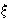i) to maximize his utility ui(x, yi) from consuming the public good x and private consumption yi, taking contributions of others as given (x-i). The consumer's problem can be then written as follows :

max ui(x, yi) = ui(x-i +i, yi) subject to constraintsi, yi0 and to p.i + yim,
where p denotes the price of one unit of the public good and m denotes the value of i-th person initial endowment or income.

First order conditions: MRSip, and MRSi = p if> 0.

Graphical Illustration of First Order Conditions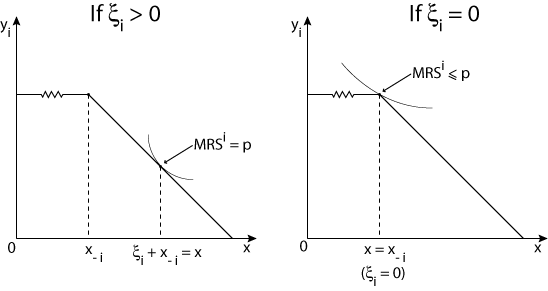Numerical Example

Suppose a unit of public good costs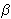and the consumer i has a utility function of the following form:

ui(x, yi) = yi +ilog x for all i = 1,...,n.
Then MRSi =i / x
Let A =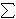ii and* = max {i | i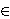N }.

Pareto Efficiency:MRSi = p
i.e.,(i / x) =(1 / x) A =x´ = A /, where x´ is the Pareto efficient outcome.

Market Outcome:

MRSip =, for all i
i.e.,i / xp, for all i
i.e., xi / p, for all i.

Let's examine when an idividual purchases positive amount of public good
MRSi = p ifi > 0, i.e., x =i /.
From this follows thati = 0 ifi <* = max {i | iN }, and xm =* /, where xm denotes the market outcome.

Note that* << A and therefore, xm << x´, meaning that the market outcome is severly inefficient.